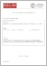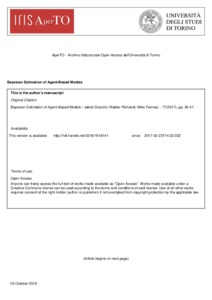# Bayesian estimation of agent-based models

Grazzini, Jakob and Richiardi, Matteo G and Tsionas, Mike (2017) 'Bayesian estimation of agent-based models.' Journal of Economic Dynamics and Control, 77. 26 - 47. ISSN 0165-1889Preview
Text
AB-v58.pdf - Accepted Version

Download (606kB) | Preview

## Abstract

We consider Bayesian inference techniques for agent-based (AB) models, as an alternative to simulated minimum distance (SMD). Three computationally heavy steps are involved: (i) simulating the model, (ii) estimating the likelihood and (iii) sampling from the posterior distribution of the parameters. Computational complexity of AB models implies that efficient techniques have to be used with respect to points (ii) and (iii), possibly involving approximations. We first discuss non-parametric (kernel density) estimation of the likelihood, coupled with Markov chain Monte Carlo sampling schemes. We then turn to parametric approximations of the likelihood, which can be derived by observing the distribution of the simulation outcomes around the statistical equilibria, or by assuming a specific form for the distribution of external deviations in the data. Finally, we introduce Approximate Bayesian Computation techniques for likelihood-free estimation. These allow embedding SMD methods in a Bayesian framework, and are particularly suited when robust estimation is needed. These techniques are first tested in a simple price discovery model with one parameter, and then employed to estimate the behavioural macroeconomic model of De Grauwe (2012), with nine unknown parameters.

Item Type: Article Agent-based, Estimation, Bayes, Approximate Bayesian computation, Likelihood H Social Sciences > HB Economic Theory Faculty of Social Sciences > Institute for Social and Economic Research Elements 03 Oct 2018 11:31 05 Feb 2019 02:00 http://repository.essex.ac.uk/id/eprint/23189

### Actions (login required)View Item